﻿ 一种耦合CFD修正的螺旋桨快速设计方法Download PDF文章快速检索 高级检索

A quick design method of propeller coupled with CFD correction
GUO Jiahao, ZHOU Zhou, FAN Zhongyun
School of Aeronautics, Northwestern Polytechnical University, Xi'an 710072, China
Abstract: The propeller design method based on the blade element momentum theory and the vortex theory has the problem of thrust deviation and cannot guarantee high efficiency due to the difference of the aerodynamic force of blade element between the design and the real situations. In order to solve this problem, the design is corrected by the numerical simulation of the propeller. Assuming that aerodynamic force of blade element along the radial direction is constant, the aerodynamic force can be inversely solved by numerical simulation results. Then the propeller is redesigned by the obtained aerodynamic force to establish a quick design method of propeller. The results show that the proposed design method can satisfy the design thrust in the small propeller design for solar energy UAV, and improve propeller efficiency by 2.75% compared with the traditional design method. And the efficiency of the propeller is further improved by 3.95% compared with the traditional design method after optimizing the airfoil of the propeller. Moreover, this method only needs to perform a small amount of CFD calculation, and the design cycle is shorter than directly using the numerical simulation to optimize the chord length and the twist angle distribution of the propeller.
Keywords: solar energy UAV    propeller    blade element momentum theory    multiple reference frames    surrogate model    optimization design    high efficiency

1 传统螺旋桨设计方法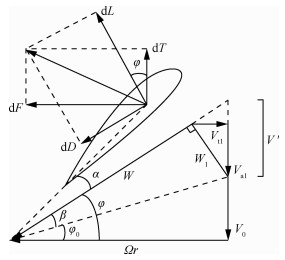图 1 螺旋桨叶素受力分析 Fig. 1 Force analysis of propeller blade element

1) 确定桨叶数NB，螺旋桨半径R，将桨叶划分为N个截面，并确定各截面翼型。

2) 根据设计拉力T及设计状态求解诱导螺距V′。

 $\mathit{\Gamma }\left( r \right) = \frac{{4{\rm{ \mathsf{ π} }}r}}{{{N_{\rm{B}}}}}{V_{{\rm{t1}}}}$ （1）

 ${\rm{d}}T = \rho \mathit{\Gamma }\left( r \right)\left( {\mathit{\Omega }r - {V_{{\rm{t}}1}}} \right){\rm{d}}r$ （2）

 ${\rm{d}}T = \frac{{4{\rm{ \mathsf{ π} }}r\rho }}{{{N_{\rm{B}}}}}\left( {{V_0} + V'{{\cos }^2}\varphi } \right)V'{\cos ^2}\varphi {\rm{d}}r$ （3）

 $T = \int_{{R_{\min }}}^{{R_{\max }}} f \frac{{4{\rm{ \mathsf{ π} }}r\rho }}{{{N_{\rm{B}}}}}\left( {{V_0} + V'{{\cos }^2}\varphi } \right)V'{\cos ^2}\varphi {\rm{d}}r$ （4）

 $f = \frac{2}{{\rm{ \mathsf{ π} }}}\arccos \exp \left[ { - \frac{{{N_{\rm{B}}}\left( {R - r} \right)}}{{2r\tan \varphi }}} \right]$ （5）

 $\mathit{\Gamma }\left( r \right) = f\frac{{4{\rm{ \mathsf{ π} }}r}}{{{N_{\rm{B}}}}}V'\cos \varphi \sin \varphi$ （6）

3) 求解r处截面的弦长b及扭转角θ

 ${\rm{d}}L = \rho \mathit{\Gamma }\left( r \right)W{\rm{d}}r$ （7）

 ${\rm{d}}L = \frac{1}{2}\rho {W^2}{C_L}b{\rm{d}}r$ （8）

 $\mathit{\Gamma }\left( r \right) = \frac{1}{2}W{C_L}b$ （9）

 $\theta = \alpha + \varphi$ （10）

4) 重复求解，得到所有截面几何信息。

5) 对弦长b及扭转角θ分布进行光顺处理。

 $\begin{array}{l} p = {\left( {1 - t} \right)^4}{p_0} + 4{\left( {1 - t} \right)^3}t{p_1} + \\ \;\;\;\;\;\;6{\left( {1 - t} \right)^2}{t^2}{p_2} + 4(1 - t){t^3}{p_3} + {t^4}{p_4} \end{array}$ （11）

 ${\rm{d}}T = \frac{1}{2}\rho {W^2}b\left( {{C_L}\cos \varphi - {C_D}\sin \varphi } \right){\rm{d}}r$ （12）
 ${\rm{d}}F = \frac{1}{2}\rho {W^2}b\left( {{C_L}\sin \varphi + {C_D}\cos \varphi } \right){\rm{d}}r$ （13）
 $T = \int_{{R_{\min }}}^{{R_{\max }}} {{N_{\rm{B}}}} {\rm{d}}T$ （14）
 $M = \int_{{R_{\min }}}^{{R_{\max }}} {{N_{\rm{B}}}} r{\rm{d}}F$ （15）
 $\eta = \frac{{T{V_0}}}{{\mathit{\Omega }M}}$ （16）

2 数值模拟方法验证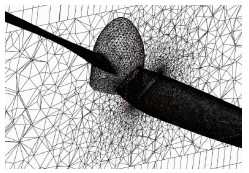图 2 局部网格示意图 Fig. 2 Schematic diagram of local mesh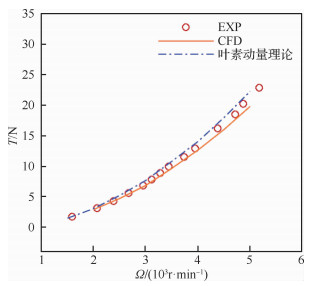图 3 拉力对比 Fig. 3 Comparison of thrust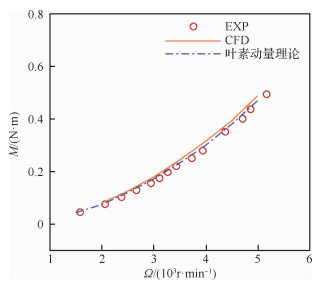图 4 扭矩对比 Fig. 4 Comparison of torque
3 基于传统设计方法的螺旋桨设计 3.1 设计状态

3.2 设计结果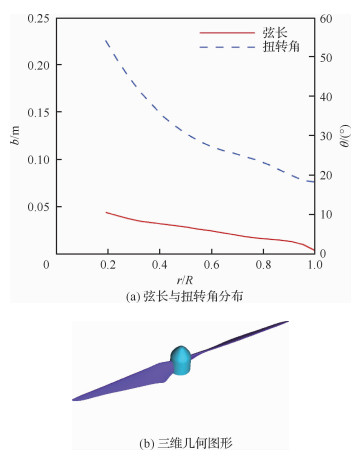图 5 螺旋桨设计结果 Fig. 5 Design results of propeller

 方法 拉力/N 扭矩/(N·m) 效率/% 设计 9.871 0.610 74.402 CFD 8.793 0.576 70.188
4 耦合CFD求解的螺旋桨快速设计

4.1 给定叶素气动力的螺旋桨设计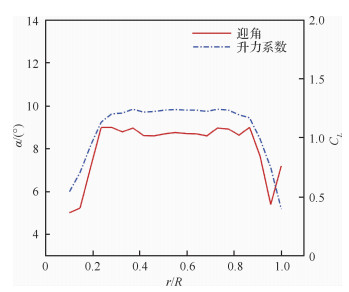图 6 迎角及升力系数径向分布 Fig. 6 Radial distribution of angle of attack and lift coefficient

4.2 根据CFD结果反解桨叶素气动力

1) 将桨叶划分为N个截面，根据设计拉力T及设计状态求解诱导螺距V′，具体步骤同传统设计方法。

2) 求解最大升阻比对应的迎角α

 $\alpha = \frac{1}{N}\sum\limits_{i = 1}^N {{\alpha _i}}$ （17）

3) 已知螺旋桨总拉力T、扭矩M，根据式(12)、式(13)，求得满足要求的升力系数CL及阻力系数CD

4.3 耦合CFD修正的螺旋桨设计方法

1) 根据给定的设计状态，采用传统螺旋桨设计方法，计算得到初始螺旋桨几何形状(弦长及扭转角分布)。

2) 由于设计采用的叶素气动力与真实情况存在差异，为保证螺旋桨效率最高，需找到真实情况叶素截面最大升阻比对应的迎角。因此，在初始几何外形的基础上，将桨叶的扭转角整体调整“±θ0”，生成n个螺旋桨外形。接着，采用CFD分别计算其拉力及扭矩，并反解其对应的桨叶叶素气动力。选取升阻比最大的气动力数据。

3) 根据选定的数据，通过给定截面气动力的螺旋桨设计方法，设计新的螺旋桨。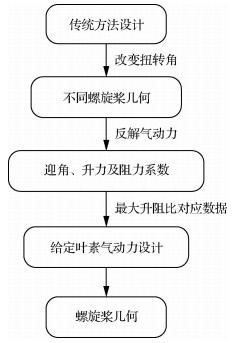图 7 螺旋桨快速设计流程 Fig. 7 Rapid design process of propeller
4.4 设计结果

 扭转角/(°) CFD结果 反解得到的气动力 拉力/N 扭矩/(N·m) 迎角/(°) 升力系数 升阻系数 升阻比 0 8.793 0.576 8.402 1.032 0.057 2 18.042 -1 8.396 0.539 7.521 0.983 0.050 0 19.660 -2 7.925 0.500 6.663 0.926 0.044 8 20.670 -3 7.412 0.463 5.819 0.864 0.041 2 20.971 -4 6.840 0.423 4.994 0.796 0.038 0 20.947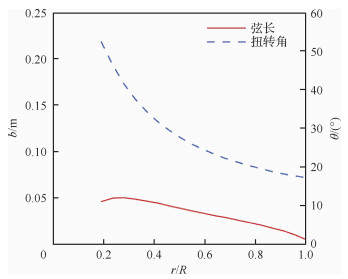图 8 新设计方法所得弦长与扭转角分布 Fig. 8 Distribution of chord length and twist angle from new design method

 设计方法 拉力/N 扭矩/(N·m) 效率/% 传统方法 8.793 0.576 70.188 新方法 9.756 0.622 72.116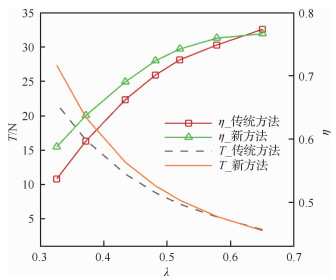图 9 传统和新设计方法螺旋桨性能对比 Fig. 9 Comparison of propeller performances between origin and new design methods
5 考虑翼型优化的螺旋桨设计方法

5.1 优化方法

5.2 优化目标及约束

 $C_D^\prime = \left( {{C_{D1}} + {C_{D2}} + {C_{D3}}} \right)/3$ （18）

5.3 翼型优化结果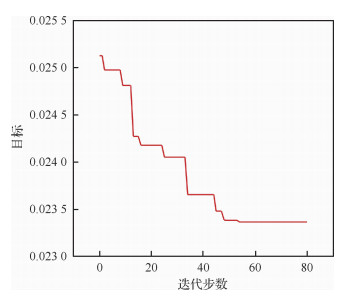图 10 优化收敛曲线 Fig. 10 Optimizing convergence curve

 翼型 平均升力系数 平均阻力系数 升阻比 基础翼型 0.943 0.026 6 35.451 优化翼型 0.974 0.023 4 41.624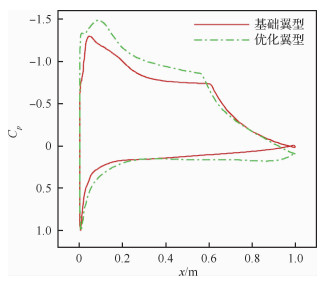图 11 优化前后翼型压力分布对比(Re=50 000) Fig. 11 Comparison of pressure distributions around airfoil before and after optimization (Re=50 000)
5.4 螺旋桨优化设计流程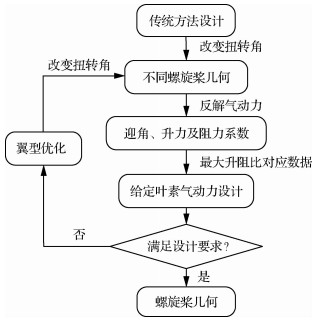图 12 考虑翼型优化的螺旋桨快速设计流程 Fig. 12 Propeller rapid design process considering airfoil optimization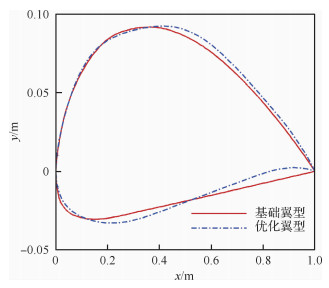图 13 优化前后翼型外形对比 Fig. 13 Comparison of airfoil shapes before and after optimization
5.5 螺旋桨性能对比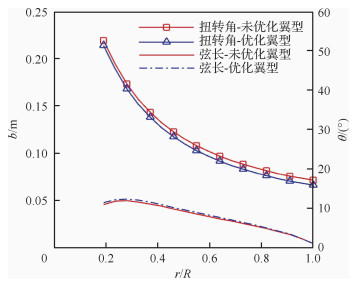图 14 优化翼型前后设计结果对比 Fig. 14 Comparison of design results before and after airfoil optimization

 设计方法 拉力/N 扭矩/(N·m) 效率/% 传统方法 8.793 0.576 70.188 新方法 9.756 0.622 72.116 新方法+优化翼型 9.743 0.614 72.958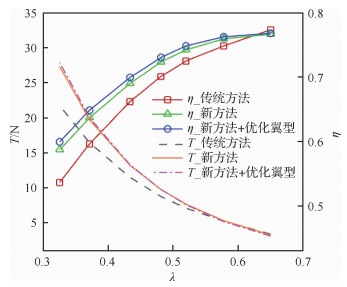图 15 不同设计方法螺旋桨性能对比 Fig. 15 Comparison of propeller performances among different design methods
6 结论

1) 传统螺旋桨设计方法存在一定缺陷。首先，设计得到的螺旋桨其真实的拉力与目标拉力存在一定偏差; 其次，不能保证真实情况下桨叶叶素截面的升阻比最大，因此不能保证设计螺旋桨的高效率。

2) 将设计与实际情况叶素气动力的差异，以及真实情况下如展向流动、桨叶桨根及桨尖处的三维效应带来的影响，归结于螺旋桨桨叶气动力的改变。提出了根据给定气动力进行螺旋桨设计的方法。假设桨叶叶素截面最大升阻比对应的气动力沿径向不变，通过整体改变螺旋桨扭转角，生成多个螺旋桨构型，再通过CFD计算及反解气动力，可得到升阻比最大的气动力数据。根据该数据进行螺旋桨的再设计，可得到高效率的螺旋桨。

3) 经过实例的对比分析，文中所建耦合CFD求解的螺旋桨设计方法，一方面能够较好地满足设计拉力要求，另一方面相比于传统设计方法，设计得到的螺旋桨效率提高了2.75%。而采用代理优化的方法对螺旋桨翼型进行优化后，相比于传统设计方法螺旋桨的效率可进一步提高3.95%。此外，该方法只需进行少量的CFD计算即可，计算量远小于直接采用数值模拟优化螺旋桨弦长及扭转角分布的方法。

  LARRABEE E E. Practical design of minimum induced loss propellers[C]//Wichita: Business Aircraft Meeting and Exposition, 1979.  ANGELO S D, BERARDI F, MINISCI E. Aerodynamic performances of propellers with parametric considerations on the optimal design[J]. The Aeronautical Journal, 2002, 106: 1-34. Click to display the text  项松, 王吉, 张利国, 等. 一种高效率螺旋桨设计方法[J]. 航空动力学报, 2015, 30(1): 136-141. XIANG S, WANG J, ZHANG L G, et al. A design method for high efficiency propeller[J]. Journal of Aerospace Power, 2015, 30(1): 136-141. (in Chinese) Cited By in Cnki (7) | Click to display the text  项松, 佟刚, 吴江, 等. 某型三叶螺旋桨的设计及性能试验[J]. 航空动力学报, 2016, 31(8): 1793-1798. XIANG S, TONG G, WU J, et al. Design and performance test of a kind of three-blade propeller[J]. Journal of Aerospace Power, 2016, 31(8): 1793-1798. (in Chinese) Cited By in Cnki (2) | Click to display the text  翟若岱.太阳能飞机高效率螺旋桨设计关键技术研究[D].沈阳: 沈阳航空航天大学, 2017. ZHAI R D. Research on key technology of high efficiency propeller design for solar powered aircraft[D]. Shenyang: Shenyang Aerospace University, 2017(in Chinese). Cited By in Cnki | Click to display the text  许建华, 宋文萍, 刘俊.基于NS方程的螺旋桨气动反设计与优化设计方法研究[C]//第十五届全国计算流体力学会议, 2012: 727-731. XU J H, SONG W P, LIU J. Investigation of inverse design and optimization design method for propellers based on Navier-Stokes equations[C]//The 15th National Conference on Computational Fluid Dynamics, 2012: 727-731(in Chinese).  梁撑刚, 郁新华, 龚军锋. 一种无人机螺旋桨的快速优化设计方法[J]. 航空计算技术, 2017, 47(2): 76-79. LIANG C G, YU X H, GONG J F. A quick approach to optimum design of UAV propeller[J]. Aeronautical Computing Technique, 2017, 47(2): 76-79. (in Chinese) Cited By in Cnki | Click to display the text  焦俊, 宋笔锋, 张玉刚, 等. 高空飞艇螺旋桨优化设计与气动性能车载试验[J]. 航空动力学报, 2017, 23(1): 196-202. JIAO J, SONG B F, ZHANG Y G, et al. Optimal design and truck-mounted testing of aerodynamic performance for the propeller of high altitude airship[J]. Journal of Aerospace Power, 2017, 23(1): 196-202. (in Chinese) Cited By in Cnki | Click to display the text  刘芳, 杜绵银, 岳良明, 等.高空低雷诺数高效螺旋桨设计[C]//第三届中国航空科学技术大会论文集, 2017: 15-21. LIU F, DU M Y, YUE L M, et al. High efficiency propeller design at low-Reynolds number and high-altitude[C]//The 3rd China Aeronautical Science and Technology Conference, 2017: 15-21(in Chinese).  KWON H, YI S, KIM T, et al. Aerodynamic performances of propellers with parametric considerations on the optimal design[C]//31st AIAA Applied Aerodynamics Conference. Reston, VA: AIAA, 2013.  MORGADO J, ABDOLLAHZADEH M, SILVESTRE M A R, et al. High altitude propeller design and analysis[J]. Aerospace Science and Technology, 2015, 45: 398-407. Click to display the text  YONEZAWA K, ABE K, SUNADA S, et al. Propeller design and loss mechanisms in low-Reynolds-number flows[J]. Journal of Propulsion and Power, 2016, 32(6): 1378-1385. Click to display the text  刘沛清. 空气螺旋桨理论及其应用[M]. 北京: 北京航空航天大学出版社, 2006. LIU P Q. Theory and application of airscrew[M]. Beijing: Beihang University Press, 2006. (in Chinese)  程晓亮, 李杰. 螺旋桨滑流对机翼气动特性影响的方法研究[J]. 科学技术与工程, 2011, 11(14): 3229-3235. CHENG X L, LI J. Unsteady computational method for the propeller/wing interaction[J]. Science Technology and Engineering, 2011, 11(14): 3229-3235. (in Chinese) Cited By in Cnki (4) | Click to display the text  王红波, 祝小平, 周洲, 等. 太阳能无人机螺旋桨滑流气动特性分析[J]. 西北工业大学学报, 2015, 33(6): 910-920. WANG H B, ZHU X P, ZHOU Z, et al. Aerodynamic investigation on propeller slipstream flows for solar powered airplanes[J]. Journal of Northwestern Polytechnical University, 2015, 33(6): 910-920. (in Chinese) Cited By in Cnki (3) | Click to display the text  王科雷, 祝小平, 周洲, 等. 低雷诺数分布式螺旋桨滑流气动影响[J]. 航空学报, 2016, 37(9): 2669-2678. WANG K L, ZHU X P, ZHOU Z, et al. Distributed electric propulsion slipstream aerodynamic effects at low Reynolds number[J]. Acta Aeronautica et Astronautica Sinica, 2016, 37(9): 2669-2678. (in Chinese) Cited By in Cnki (1) | Click to display the text  韩忠华. Kriging模型及代理优化算法研究进展[J]. 航空学报, 2016, 37(11): 3197-3225. HAN Z H. Kriging surrogate model and its application to design optimization:A review of recent progress[J]. Acta Aeronautica et Astronautica Sinica, 2016, 37(11): 3197-3225. (in Chinese) Cited By in Cnki (5) | Click to display the text  SACKS J, WELCH W J, MITCHELL T J, et al. Design and analysis of computer experiments[J]. Statistical Science, 1989, 4(4): 409-423. Click to display the text  JONES D R, SCHONLAU M, WELCH W J. Efficient global optimization of expensive black-box functions[J]. Journal of Global Optimization, 1998, 13(4): 455-492. Click to display the text  MORGADO J, VIZINHO R, SILVESTRE M A R, et al. XFOIL vs CFD performance predictions for high lift low Reynolds number airfoils[J]. Aerospace Science and Technology, 2016, 52: 207-214. Click to display the text  KULFAN B M. Universal parametric geometry representation method[J]. Journal of Aircraft, 2008, 45(1): 142-158. Click to display the text
http://dx.doi.org/10.7527/S1000-6893.2019.23216

0

#### 文章信息

GUO Jiahao, ZHOU Zhou, FAN Zhongyun

A quick design method of propeller coupled with CFD correction

Acta Aeronautica et Astronautica Sinica, 2020, 41(2): 123216.
http://dx.doi.org/10.7527/S1000-6893.2019.23216